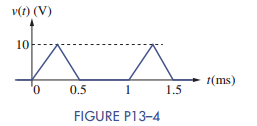### Create an Account

Home / Questions / A half-wave rectified sine wave has an amplitude of 169 V and a fundamental frequency of 6...

# A half-wave rectified sine wave has an amplitude of 169 V and a fundamental frequency of 60 Hz. Use the results in Figure 13–4 to write an expression for the first four nonzero terms in the Fourier

A half-wave rectified sine wave has an amplitude of 169 V and a fundamental frequency of 60 Hz. Use the results in Figure 13–4 to write an expression for the first four nonzero terms in the Fourier series. Use MATLAB to plot the amplitude spectrum of the signal.May 24 2020 View more View LessSubscribe To Get Solution